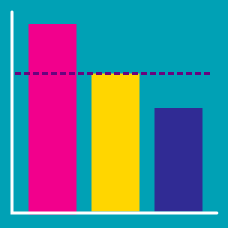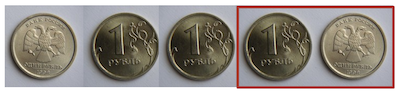Probability

# Expected Value - Problem SolvingWhat is the expected number of coin flips we must make, before we see a Head followed immediately by a Tail?

Let $X, \ Y, \ Z$ be random variables over $\mathbb{Z}_{3}, \ \mathbb{Z}_{3}, \ \mathbb{Z}_{4},$ respectively, where $\mathbb{Z}_n$ denotes $\{1, 2, \dots, n\}.$ If $P_{X, Y, Z}(x, y, z) = \frac{xy}{144},$ what is the greatest integer less than or equal to $E[X + Y | Z]?$

Suppose $E[X|Y=0] = 1$ and $E[X|Y=1] = 9.$ When $P(Y=0) = 0.2$ and $P(Y=1) = 0.8,$ what is $E[X]?$

Given $Z=9,$ if $X$ and $Y$ are uniform random variables over $\{0, 1, \dots, 9\},$ what is $E[ X + Y | Z=9 ] ?$

When flipping a fair coin repeatedly, what is the expected value of the number of trials needed to get two tails in a row?

×